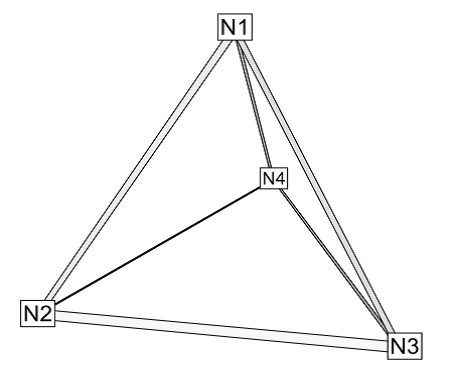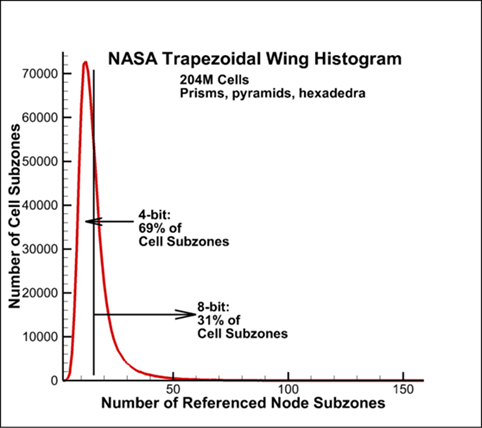# Serendipitous Side Effect of SZL Technology

Blog by Dr. Scott Imlay, Chief Technical Officer at Tecplot, Inc.

“There’ll always be serendipity involved in discovery.”

— Jeff Bezos

In previous blogs, I’ve discussed the SZL (Subzone Load-on-Demand) technology we are using to visualize a trillion cells. In this blog, I’d like to discuss a serendipitous side effect of the SZL technology. It is fairly easy to compress finite-element node maps. It is not entirely serendipitous—we worked hard to implement the compression—but it is so much easier to do when working with the SZL technology.

### Uncompressed Node-map

To understand the compression, let’s first review the uncompressed node-map. In finite-element data, the elements (synonymous with cells) are defined by a list of node-numbers for the nodes at the corners of the elements. So, for example, four node numbers are needed to define a tetrahedral element.Figure 1. Four node numbers are needed to define a tetrahedral element.

### General Finite-element Grid

In a general finite-element grid, no spatial organization of the node-numbers is required. The values of N1 through N4 may be all over the map, from 1 to the number of nodes in the actual grid. In Tecplot 360 plt files, the node-map is the list of 32-bit integers specifying the corner node numbers for each element in the grid:

N11,N21,N31,N41

N12,N22,N32,N42,N52

N1NumElems,N2NumElems,N3NumElems,N4NumElsms

If there are a million elements, this is four million 32-bit (four byte) numbers, or roughly 4MB of data.

### Spatially Cohesive Subzones

With SZL files, the cells are grouped in spatially cohesive cell subzones of 256 cells or less, and the nodes are grouped in spatially cohesive node subzones of 256 nodes or less. The node numbers are now given in terms of a node subzone number, S_n, and a node number (in the range 1 to 256), of the desired node in the node subzone. Now, instead of four 32-bit integers for each cell we store four 24-bit node-subzone numbers, S, and four 8-bit node-subzone node numbers, n. We store these as two consecutive lists:

S11,S21,S31,S41

S12,S22,S32,S42

S1NumElems,S2NumElems,S3NumElems,S4NumElsms

n11,n21,n31,n41

n12,n22,n32,n42

n1NumElems,n2NumElems,n3NumElems,n4NumElsms

### Node Subzones Referenced by Cell Subzones

So far we’ve just broken the 32-bit node numbers into a 24-bit node-subzone number and an 8-bit node-subzone node number. We haven’t compressed anything, we’ve just reorganized it. The compression comes with the realization that the number of node-subzones needed for each cell subzone is far less than the 16.8 million possibilities given in a 24-bit number. In fact, most cell subzones reference far fewer than 256 node subzones. For example, consider the NASA Trapezoidal Wing we’ve worked with in previous blogs. The following figure is a histogram of the number of node subzone referenced by the cell subzones.Histogram of the number of node subzone referenced by the cell subzones.

For this grid, 69% of the cell subzones reference 16 or fewer node subzones and the remaining 31% reference more than 16 but fewer than 257 node subzones. For this grid we don’t need a 24-bit integer to specify the node-subzone number – an 8-bit integer should do.

### Saving Memory and Disk Space

To take advantage of this fact, we modify our previous node-map to include a list of the needed node-subzones, S, at the top and replace the old cell-by-cell references node-subzone numbers with reduced precision offsets, O, into the new list of referenced node subzones. For Trapezoidal wing case, for 69% of the cell subzones the offsets can be 4-bit integers and for the remaining 31% of the cell subzones the offsets can be 8-bit integers. Compared to the 24-bit integers we had originally, this saves a huge amount of memory and disk space. The resulting node-map for a subzone looks like this:

S1, S2, S3, S4, S5, …

O11,O21,O31,O41

O12,O22,O32,O42

O1256,O2256,O3256,O4256

n11,n21,n31,n41

n12,n22,n32,n42

n1256,n2256,n3256,n4256

The list of node-subzone numbers requires additional space, but there is only a few of them. The additional space for this list is more than offset by the reduction in precision of the 1024 offsets.

### Node-map compression is a WIN, WIN, WIN!

So how well does it work? It turns out to work quite well. For the Trapezoidal wing case mentioned above, the node-map is compressed by 57% and, because the node-map is such a large portion of the data file, this results in a 37% compression overall. Finally, the time to generate a slice with SZL data is actually 13% faster with compression. As impressive as these results are, for many files the compression works even better with overall compression of up to 55%. Node-map compression is a WIN, WIN, WIN!